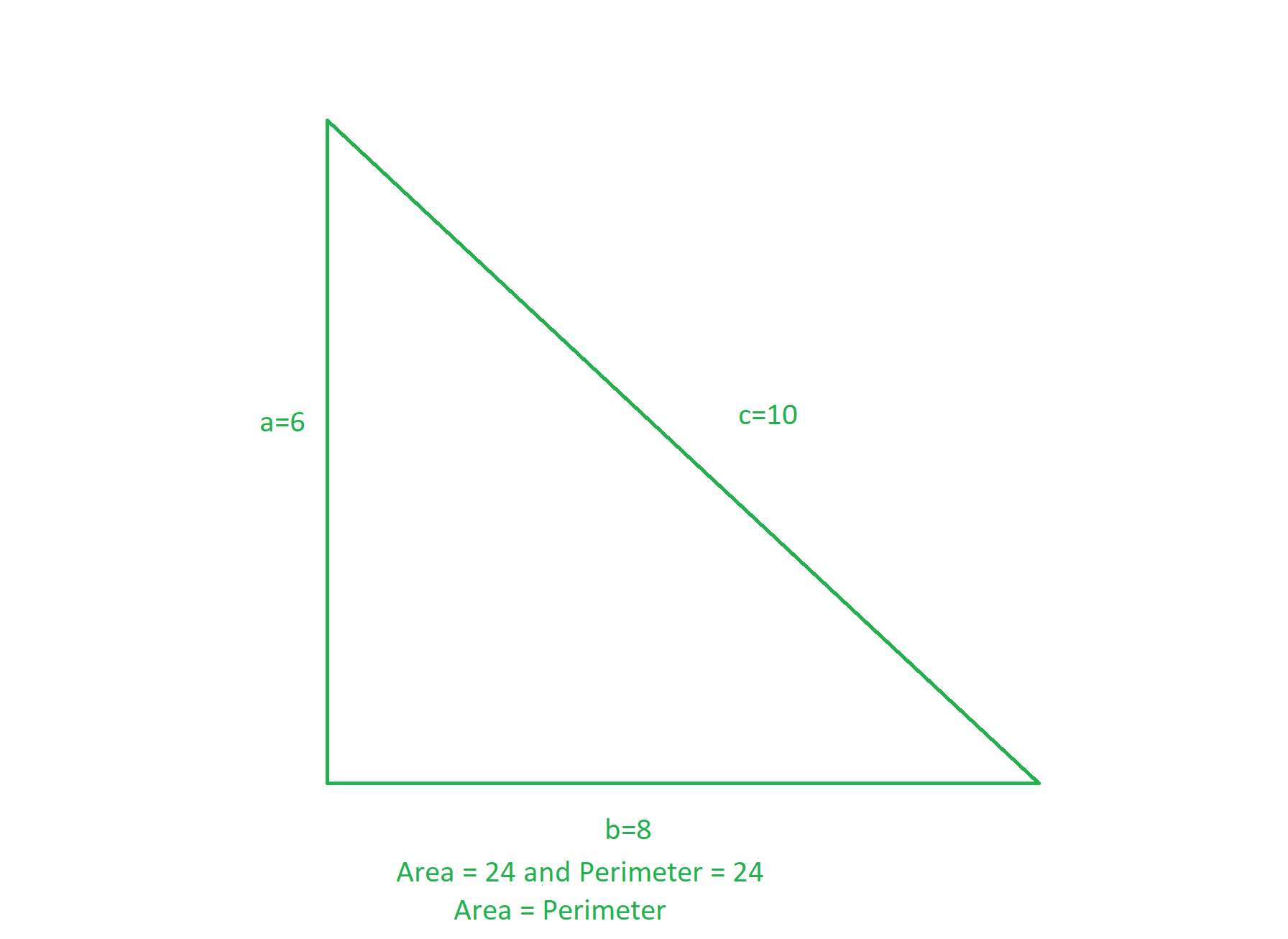Related Articles
Program to find all possible triangles having same Area and Perimeter
• Difficulty Level : Easy
• Last Updated : 16 Dec, 2020

The task is to find all possible triangles having the same perimeter and area.

Examples:The triangle having sides (6, 8, 10) have the same perimeter (= (6 + 8 + 10) = 24) and area (= 0.5 * 6 * 8 = 24).

Approach: The idea is based on the observation from Heron’s Formula. Below are the observations:

Let the sides of the triangle be a, b, c.
Perimeter(P) = a + b + c
Area(A) using Heron’s Formula:where s = (a + b + c) / 2

Experimental Observation:

We know that:
4 * s2 = s * (s – a) * (s – b) * (s – c)
=> 4 * s = (s – a) * (s – b) * (s – c)
=> 2 * 2 * 2 * 4 * s = 2 * (s – a) * 2 * (s -b) * 2 * (s – c)
=> 16 * (a + b + c) = (- a + b + c) * (a – b + c) * (a + b – c)

Due to this condition:
Max value of (- a + b + c), (a – b + c), (a + b – c) is as follows:
(- a + b + c) * (a – b + c) * (a + b – c) ≤ 16 * 16 * 16
=> 16 * (a + b + c) ≤ 16 * 16 * 16
=> (a + b + c) ≤ 256

From the above equation, the sum of sides of the triangle doesn’t exceed 256 whose perimeter of triangle and area of the triangle can be the same. Therefore, the idea is to iterate three nested loops over the range [1, 256] and print those triplets of sides having the same area and perimeter.

Below is the implementation of the above approach:

## C++

 // C++ program for the above approach #include using namespace std; // Function to print sides of all the// triangles having same perimeter & areavoid samePerimeterAndArea(){    // Stores unique sides of triangles    set > se;     // i + j + k values cannot exceed 256    for (int i = 1; i <= 256; ++i) {         for (int j = 1; j <= 256; ++j) {             for (int k = 1; k <= 256; ++k) {                 // Find the value of 2 * s                int peri = i + j + k;                 // Find the value of                // 2 * ( s - a )                int mul1 = -i + j + k;                 // Find the value of                // 2 * ( s - b )                int mul2 = i - j + k;                 // Find the value of                // 2 * ( s - c )                int mul3 = i + j - k;                 // If triplets have same                // area and perimeter                if (16 * peri == mul1 * mul2 * mul3) {                     // Store sides of triangle                    vector<int> v = { i, j, k };                     // Sort the triplets                    sort(v.begin(), v.end());                     // Inserting in set to                    // avoid duplicate sides                    se.insert(v);                }            }        }    }     // Print sides of all desired triangles    for (auto it : se) {        cout << it << " "             << it << " "             << it << endl;    }} // Driver Codeint main(){    // Function call    samePerimeterAndArea();     return 0;}

## Python3

 # Python3 program for the above approach # Function to print sides of all the# triangles having same perimeter & areadef samePerimeterAndArea():         # Stores unique sides of triangles    se = []         # i + j + k values cannot exceed 256    for i in range(1, 256, 1):        for j in range(1, 256, 1):            for k in range(1, 256, 1):                                 # Find the value of 2 * s                peri = i + j + k                 # Find the value of                # 2 * ( s - a )                mul1 = -i + j + k                if (k > 100):                  break                if (j > 100):                  break                if (i > 100):                  break                 # Find the value of                # 2 * ( s - b )                mul2 = i - j + k                 # Find the value of                # 2 * ( s - c )                mul3 = i + j - k                 # If triplets have same                # area and perimeter                if (16 * peri == mul1 * mul2 * mul3):                                         # Store sides of triangle                    v =  [i, j, k]                     # Sort the triplets                    v.sort(reverse = False)                     # Inserting in set to                    # avoid duplicate sides                    se.append(v)                    se.sort(reverse = False)     # Print sides of all desired triangles    temp = []    temp.append(se)    temp.append(se)    temp.append(se)    temp.append(se)    temp.append(se)    for it in temp:        print(it, it, it) # Driver Codeif __name__ == '__main__':         # Function call    samePerimeterAndArea()     # This code is contributed by ipg2016107
Output:
5 12 13
6 8 10
6 25 29
7 15 20
9 10 17

Time Complexity: O(2563)
Auxiliary Space: O(2563)

Attention reader! Don’t stop learning now. Get hold of all the important mathematical concepts for competitive programming with the Essential Maths for CP Course at a student-friendly price. To complete your preparation from learning a language to DS Algo and many more,  please refer Complete Interview Preparation Course.

My Personal Notes arrow_drop_up